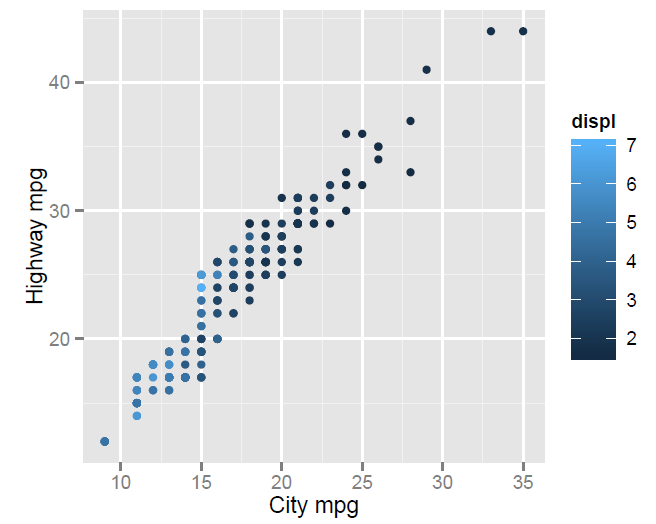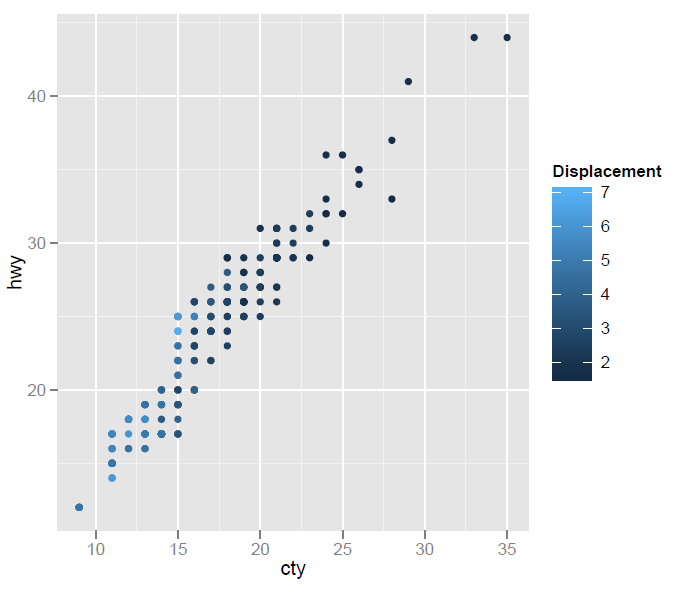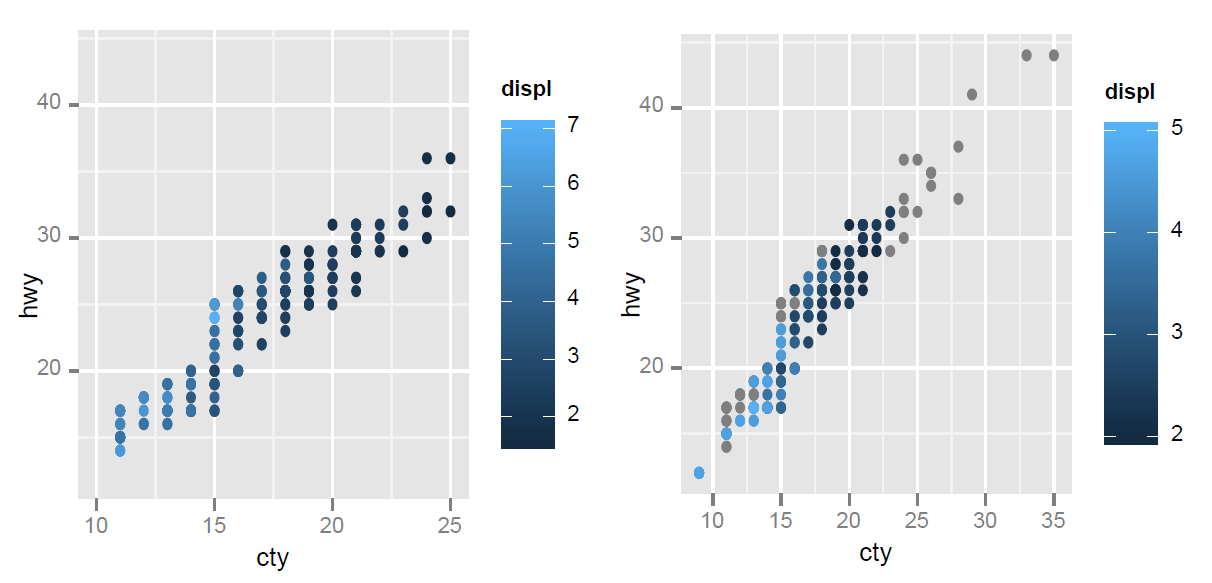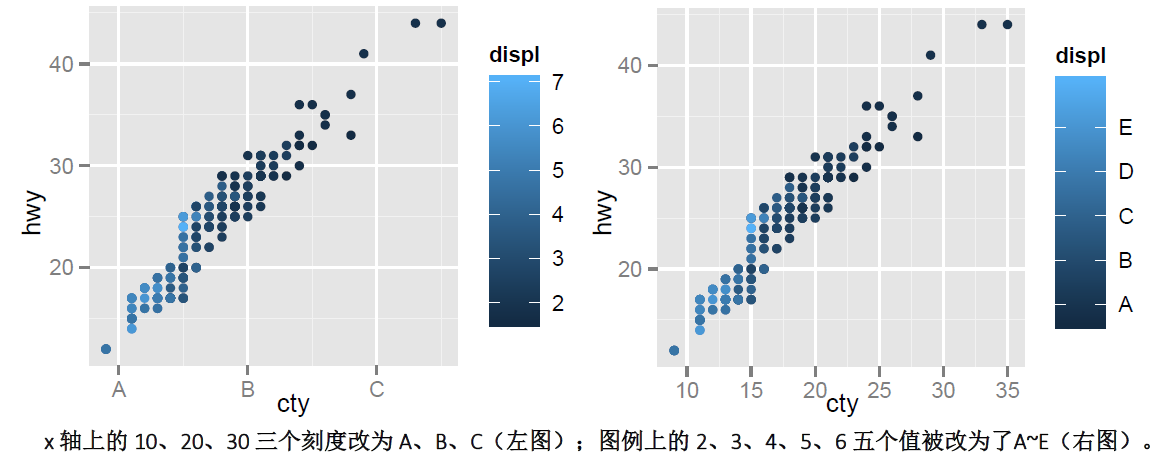# ggplot2学习笔记之标度scale

• scale_x_XXX、scale_y_XXX 是控制x、y 轴的标度，用于设置x、y 轴的显示标签、显示范围、刻度上的值等等。XXX 主要有continuous、data、datatime、discrete、log10、sqrt 等等。
• 对于图例的标度，如果图例是控制着颜色属性colour，那么使用scale_colour_XXX 可以设置这个图例的属性（比如标签、显示的值）。类似的还有scale_size_XXX、scale_fill_XXX、scale_shape_XXX 等等，具体用法以及参数请查看帮助文档。

### 1. 设置坐标轴与图例标签

• 设定坐标轴或者图例的标签：

``````p <- qplot(cty,hwy,data=mpg,colour=displ)
p + scale_x_continuous('City mpg') + scale_y_continuous('Highway mpg')
p + xlab('City mpg') + ylab('Highway mpg')
p + labs(x = ('City mpg'), y = ('Highway mpg'))``````• 设置图例显示标签：
``````p + scale_colour_gradient('Displacement')
p + labs(colour = 'Displacement')``````### 2. 参数limits

limits 可以用于固定标度定义域，即指定数据显示范围。连续型标度接受长度为2 的数值型向量；离散型接受一个字符型向量。limits 影响的是图形上显示的元素。

``````p + scale_x_continuous(limits=c(10,25))### 3. 参数breaks 和labels

breaks 控制着显示坐标轴或图例上的值，即坐标轴上应该显示哪些刻度线的值，或一个连续标度在一个图例中将被如何分段。breaks 影响显示坐标轴或图例上的元素。
labels 指定了应在断点处显示的标签。如果设置了labels，则必须同时指定breaks，只有这样两个参数才能正确匹配。

``````p + scale_x_continuous(breaks=c(10,20,30))
p + scale_colour_gradient(breaks=c(2, 3, 4, 5))````````````p + scale_x_continuous(breaks=c(10,20,30),labels=c('A','B','C'))